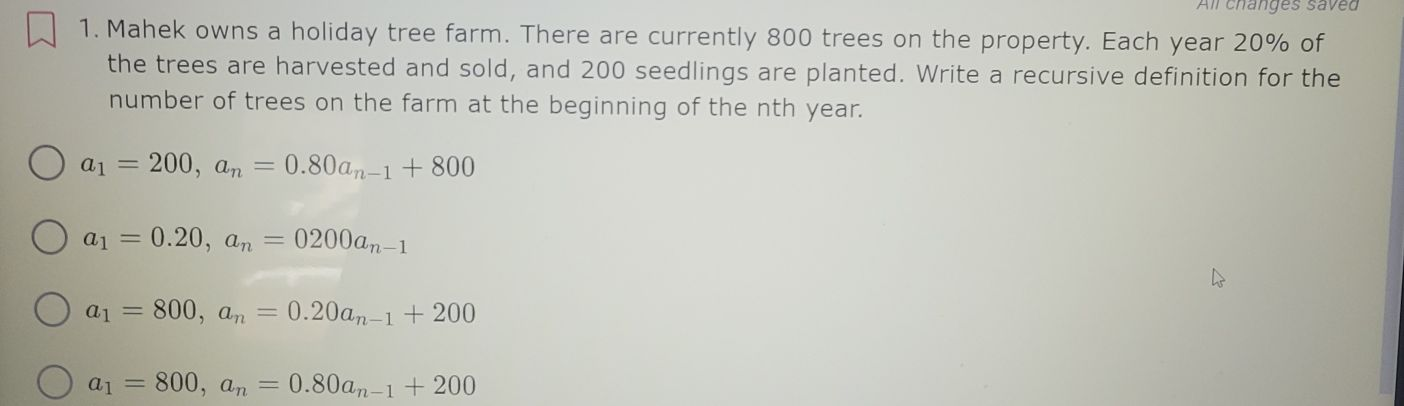### Still have math questions?

Algebra
Question1. Mahek owns a holiday tree farm. There are currently $$800$$ trees on the property. Each year $$20 \%$$ of the trees are harvested and sold, and $$200$$ seedlings are planted. Write a recursive definition for the number of trees on the farm at the beginning of the nth year.

$$a _ { 1 } = 200 , a _ { n } = 0.80 a _ { n - 1 } + 800$$

$$a _ { 1 } = 0.20 , a _ { n } = 0200 a _ { n - 1 }$$

$$a _ { 1 } = 800 , a _ { n } = 0.20 a _ { n - 1 } + 200$$

$$a _ { 1 } = 800 , a _ { n } = 0.80 a _ { n - 1 } + 200$$